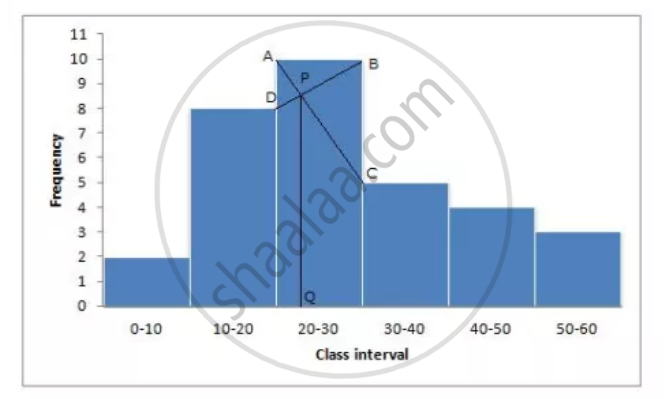# Draw a Histogram and Hence Estimate the Mode for the Following Frequency Distribution: - Mathematics

Sum

Draw a histogram and hence estimate the mode for the following frequency distribution:

 Class 0-10 10-20 20-30 30-40 40-50 50-60 Freq 2 8 10 5 4 3

#### Solution

Draw a histogram(1) In the highest rectanglr which represents modal class draw two lines AC aand BD intersecting at P.

(2) From P, draw a perpendicular to x-axis meeting at Q

(3) value of Q is the mode = 23

Concept: Concept of Median
Is there an error in this question or solution?